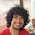### Expected Number of HH

Source: Placement Paper of one of the CS companies

Problem: A coin is tossed 10 times and the output written as a string. What is the expected number of HH? Note that in HHH, number of HH = 2

Example: In 3 tosses
HHH (2 HH)
HHT (1 HH)
HTH (0 HH)
HTT (0 HH)
THH (1 HH)
THT (0 HH)
TTH (0 HH)
TTT (0 HH)
Expected number of HH in 3 tosses = 2/8 + 1/8 + 1/8 = 0.5

1.9/4?
Because each extra coin, say ith, brings in 1/4 expected heads as i-1 coins will have 1/2 of the sequences ending in H thus 1/2*1/2(prob. of i = H) = 1/4 extra expected H
Thus for 10 coins, (10-1)/4

Please confirm if this is correct

2.This is the answer I wrote. But we were told that the answer is wrong.

3.I still feel that the answer is correct.Confirm it by running the following java program:

/*
* To change this template, choose Tools | Templates
* and open the template in the editor.
*/

package javaapplication1;

import java.math.*;
import java.util.*;
/**
*
* @author anshum
*/
public class Main {

/**
* @param args the command line arguments
*/
public static void main(String[] args) {
int numberOfHH = 0;
Random r = new Random();
for(int i = 1;i<10000000;i++)
{
for(int j=0;j<10;j++)
{
double a = r.nextDouble();
if(a<0.5)
else
{
numberOfHH++;
}
}
}
System.out.println( (double)numberOfHH/10000000.0);
// TODO code application logic here
}

}

It gives the answer as 2.25 approx

4.jaadu rocks!! Thanx for the code.
But I am sure he said that the answer is wrong. :(

Will call someone in a few days and confirm.

5.i am bad at probability, but that's exactly the solution i just thought of.

6.Just confirmed with people who made the paper and interviewed me :P

The answer 2.25 is correct. The solution I gave was not.

Let the expected number of HH for n tossed is E(n)

So, probability that an n-1 toss experiments, ends in T is 1/2.

So, E(n) = 1/2*E(n-1) + 1/2*( 1/2*(E(n-1)+1) + 1/2*(E(n-1)))
{
The first case when it ends in T.
The second case when it ends in H.

In the second case, if you get an H then, you get 1 more HH.
}
So, E(n) = E(n-1) + 1/4
E(2) we know is 1/4

So, E(n) = (n-1)/4

For n=10, E(10) = 2.25 :)

7.Sol Using Linearity of Expectations ::

let X be the random variable denoting the number of HH's.

Let X_i be the indicator random variable which takes value 1 if H's occur in ith & (i+1)th positions, and 0 otherwise.

Note that each X_i is identically distributed, hence their expected values will be same.
E[X_i]= Pr( X_i = 1 ) = 1/4.

Now, X = X_1 + X_2 + .... + X_(n-1)

By Linearity of Expectation
E[X]=E[X_1]+E[X_2]+ ... +E[X_(n-1)]
E[X] = (n-1) E[X_1].
Hence, E[X] = (n-1)/4.

8.This can be done very easily.only possible pairs in the result can be HH,HT,TH,TT.And the expected number of occurrences of these must be equal as there is no reason for precedence for one over another.Therefore ans=(n-1)/4.